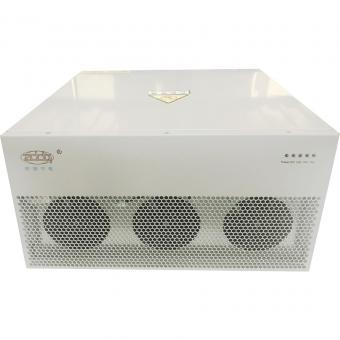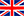English

###### How to size Active Harmonic Filter from Power Analyser Data
Dec 23,2019

The Graph below shows Harmonic Current Distortion in the form of THDi. This is hovering between 20 and 25%. Ideally, it should be less than 10% and preferably less than 8% THDi.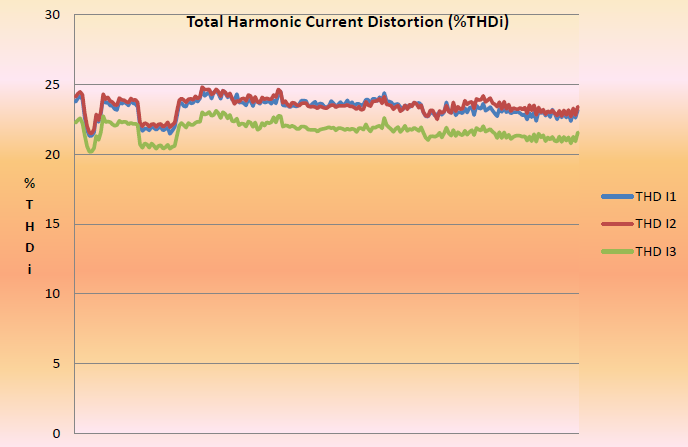The highest value of average total harmonic distortion (THDi%) across three phases was calculated from raw data. This value is 24.19%THDi and was recorded at time shown.

At the same time, average line current (Amps) across three phases was 516.83A.

Analysis of Findings
From data above, Highest Average THDi = 24.19%

At the same time, Average I RMS across three phases was 516.83A.

Taking X to be = fundamental frequency current

I RMS = √(12 + 0.24192) * x = 516.83A
1.02884 * x = 516.83
x = 516.83 / 1.02884 = 502.34A

Calculate harmonic current

I RMS = √(502.342 + Harmonic Current2) = 516.83A
502.342 + Harmonic Current2 = 267113
Harmonic Current2 = 267113 – 252345 = 14767.8A
Thus Harmonic current = 121.52A

If %THDi = 8% (An appropriate value to satisfy electrical supply utilities)
I RMS = √(502.342 + (0.08*502.34)2)
= √(252345 + 1615)
=503.94A

I RMS = √(502.342 + Harmonic Current2) = 503.94A
502.342 + Harmonic Current2 = 253955.5
Harmonic Current2 = 253955.5 – 252345.5 = 1610A
Thus Harmonic current = 40.125A

Thus – To reduce % THDi at Incomer from 24.91% to 8% requires 81.4A of harmonic filtering(121.52-40.125). Active Harmonic filters come in sizes of 60A, 120A,200A and 300A. To reduce THDi to a level of 8% would require a 120A filter.

Check performance when filter installed.

Click Active Harmonic Filter for more detials about AHF.

Blog
Hot Products
•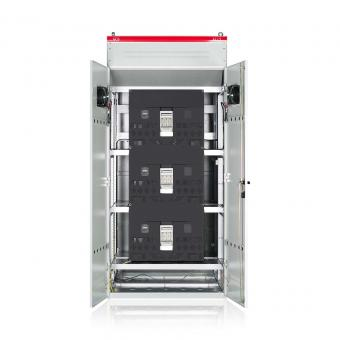#### Capacitor banks and harmonic filters. Low voltage

•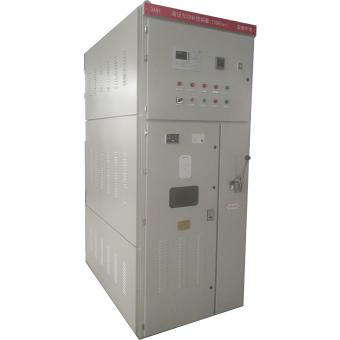#### 3.3KV Local reactive power compensation for motors,Arc Furnace

•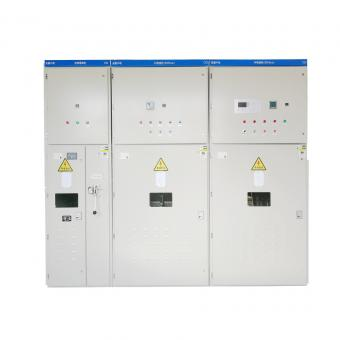#### Medium voltage Automatic Capacitor banks with harmonic filters

•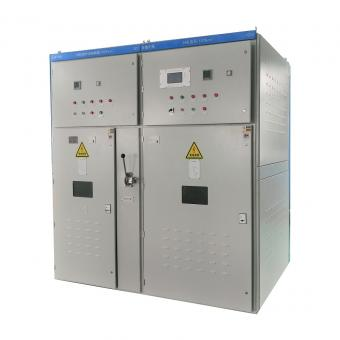#### High voltage Automatic Power Factor improvement plant for Motors VFDs

•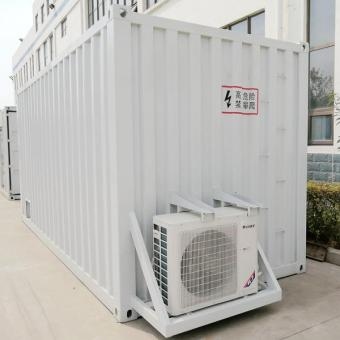#### High voltage static var generator HV SVG Statcom outdoor container

•#### Medium voltage outdoor Static Synchronous Compensator(STATCOM)

•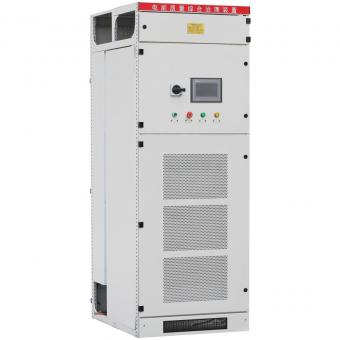•